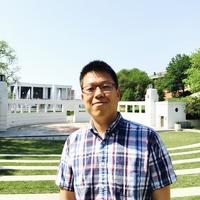# Math and Stat Profile# Shitao Liu

Mathematical and Statistical Sciences

## Associate Professor

864-656-4477
Martin Hall O228 [Office]

## Educational Background

PhD, Mathematics, University of Virginia, 2011
BS, Mathematics, Nankai University, 2006

## Research Interests

Control theory and inverse problems for partial differential equations.

## Courses Taught

MATH 9700 Analysis Bridge Course
MATH 9270 Functional Analysis
MATH 8370 Calculus of Variations and Optimal Control
MATH 8260 Partial Differential Equations
MATH 8210 Linear Analysis
MATH 4630/4640, Real Analysis I, II
MATH 4530/6530, 4540/6540 Advanced Calculus I, II
MATH 4350 Complex Variables
MATH 2060 Multivariable Calculus
MATH 1060 Calculus of Single Variable

## Selected Publications

Source;reconstruction and stability via boundary control of abstract viscoelastic systems, (with W. Green), Applicable Analysis, to appear.
Boundary observability for the viscoelastic wave equations (with W. Green and M. Mitkovski), SIAM Journal on Control and Optimization, 57 (2019), no.3, 1629--1645.
Determine a magnetic Schrodinger operator with a bounded magnetic potential from partial data in a slab (with Y. Yang), Applied Mathematics and Optimization, 83 (2018), no.1, 277--296.
A Lipschitz stable reconstruction formula for the inverse problem for the wave equation (with L. Oksanen), Transactions of the American Mathematical Society, 368 (2016), 319--335.
An inverse problem for a third order PDE arising in high-intensity ultrasound: global uniqueness and stability by one boundary measurement (with R. Triggiani), J. Inverse Ill-Posed Probl., 21 (2013), 825--869.
Recovering damping and potential coefficients for an inverse non-homogeneous second-order hyperbolic problem via localized Neumann boundary trace (with R. Triggiani), Discrete and Continuous Dynamical Systems A, 33 (2013), 5217--5252.
Recovery of the sound speed and initial displacement for the wave equation by means of a single Dirichlet boundary measurement, Evolution Equations and Control Theory, 2 (2013), no.2, 355--364.
Global uniqueness and stability in determining the damping coefficient of an inverse hyperbolic problem with non-homogeneous Dirichlet B.C. through an additional localized Neumann boundary trace (with R. Triggiani), Appl. Anal.,91 (2012), no.8, 1551--1581.
Recovering of damping coefficients for a system of coupled wave equations with Neumann B.C.: uniqueness and stability (with R. Triggiani), Chin. Ann. Math. Ser. B, 32 (2011), no.5, 669--698.
Global uniqueness in determining electric potentials for a system of strongly coupled Schr\"{o}dinger equations with magnetic potential terms (with R. Triggiani), J. Inverse Ill-Posed Probl.,19 (2011), no.2, 223-254.
Inverse problem for a structural acoustic interaction, Nonlinear Anal., 74 (2011), no.7, 2647--2662.
Global uniqueness and stability in determining the damping coefficient of an inverse hyperbolic problem with non-homogeneous Neumann B.C. through an additional Dirichlet boundary trace (with R. Triggiani), SIAM J. Math Anal., 43(2011), no.4, 1631--1666.